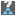• # Is the task how-much-gold well defined?

## Question related to missionHow Much Gold

I'm puzzled by the phrase "This bar appears to be made of various metal including gold, iron, copper and tin." As I read it, this means that it can contain these metals but it can contain other metals too.

So, for the first example, let us suppose that the fractions of gold, iron, copper and tin are respectively g, i, c, and t, and that x is the fraction of all other metals. This leads to a system of equations:

```g+t = 1/2
g+i = 1/3
g+c = 1/4
g+i+c+t+x = 1
```

```g = 1/24
i = 7/24
c = 5/24
t = 11/24
x = 0
```

but also the solution:

```g = 1/12
x = 1/12
t = 5/12
i = 3/12
c = 2/12
```

So, I suppose the task's story means to say that the bar does not contain any other metal except gold, iron, copper and tin. This should be fixed, I guess...